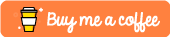Python Generators Tutorial

This tutorial was built using Python 3.6

In this tutorial I’m aiming to help demystify this concept of generators within the Python programming language. We’ll look at what generators are and how we can utilize them within our python programs.

What Are Generators?

Generators are functions that can return multiple values at different times. These are iterators that we can subsequently iterate over in a variety of different ways.

Imagine we wanted to define a function that would return double the value of whatever we first passed into it. If we wanted to do this with normal python functions we would have to do something like this:

def my_func(x):
return x*2

However this function would return the value of x doubled only once. However with generators we could make it double every time we called the next() function on this particular generator. This is where the yield keyword comes into play. You can think of yield in a similar way to how you would think of return in a normal function. Except you can call yield as many times as you would like.

Let’s define a very simple generator that will double the value of whatever we pass into it a grand total of three times, so if we pass 2 into it, by the final call we should end up with a value of 16.

def my_generator(x):
yield x*2
yield x*4
yield x*8

Complete Code Snippet

Let’s flesh this out into a full python script. In this we have our new generator function with it’s 3 yield statements, below that we declare an instance of our generator by calling mygen = my_generator(2). We then print out the next(mygen) value 3 different times to the console.

def my_generator(x):
yield x*2
yield x*4
yield x*8

mygen = my_generator(2)

print(next(mygen))
print(next(mygen))
print(next(mygen))

Output

If we were then to run this code and call the next() function 3 times on this generator, you should see the value is doubled every time. The above code should then print out the following in the console:

\$ python3.6 generators.py
4
8
16

StopIteration Error

The StopIteration error is raised when a call to next() is made and there are no subsequent values to be yielded from our generator function. If we added a 4th call to print(next(mygen)) in the previous sample then you should see an error like so print out:

\$ python3.6 generators.py
4
8
16
Traceback (most recent call last):
File "generators.py", line 12, in <module>
print(next(mygen))
StopIteration
FAIL

Infinite Generator

Let’s now improve upon our original generator function. We can implement an iterator that continues to yield values indefinitely. This will keep a reference of what value x currently is and after every yield it will double that value.

def my_generator(x):
x = x
while True:
yield x*2
x = x*2

mygen = my_generator(2)

print(next(mygen))
print(next(mygen))
print(next(mygen))
print(next(mygen))

Output

We can then call the next() function as many times as we’d desire and we would then see our original value of x double with each subsequent call to our next(mygen) function. Try change the value passed in to my_generator() and experiment with the number of times you call print(next(mygen)).

\$ python3.6 generators.py
4
8
16
32

It’s important to note that the physical limitations still apply and I’m sure you’d start to hit some form of limit for the above program if you called next() indefinitely.

Conclusion

So, in this tutorial, we looked at generators in Python! We looked at how we can build our own Python generators and subsequently use them within our own Python applications for fame and fortune!

Hopefully you found this tutorial useful! If you did or require further assistance then please let me know in the comments section below or by tweeting me @Elliot_F.